# CAT Questions | CAT Algebra Questions: Function

### CAT Verbal Ability

Functions from the perspective of CAT questions, SNAP Questions and XAT Questions have utmost importance however from other management entrance exams’ point of view the formation of the problem from this area is comparatively low. To know about the concept let us have an introduction to functions  first.

Functions for cat is a concept of algebra for cat that studies the dependence between variable quantities in the process of their change. Let’s consider one algebraic function examples, with a change in the side of a square, the area also varies. The CAT questions of how the change in the side of the square affects the area is answered by a mathematical relationship between the area of the square and the side of the square.

Let the variable x take on numeric values from the set D. A function is a rule that refers to every number x from D and one definite number y where y belongs to a set of real numbers

Here x is called the independent variable and y is known as the range of the function.

here the domain of the function is referred to by set D and the value attain by variable y will be the range of the function

Moreover, we call a variable y the value of each value of x if it as belonging to the domain where each value corresponds to a definite value of the variable y

This is written as y=f(x) where f denotes the rule by y varies with x.

## Understanding The Concepts Of Functions

Before looking at the types and example let us understand the cat function concepts and make it easy for solving algebra questions for cat So, as we know the definition of Functions accordingly

Let us consider X and Y as two non-empty subsets. A relation f from X to Y i.e. a subset of X x Y is called a function from X to Y, If

1. For each, x ϵ X there exists y ϵ Y such that (x, y) ϵ f.

2. (x, y) ϵ f and (x, z) ϵ f => y = z.

And that is the mathematical definition now let us focus on what it means. Suppose we have 2 sets, for example:

let there’s set X = {x, y, z, w} and set Y = {1, 2, 3, 4} and let us call the set M to be domain and N be codomain. Then, for each element present in A i.e. a, b, c, d there is usually a unique element corresponding to each of them in B.

Now that we have understood what conditions are necessary for functions. We can move to the basic properties and nature of functions.

Firstly, the basic notation of functions is B = f(a)

Therefore, for any quadratic equation such as B= m^2+m+5 can be re-written as f(a)= m^2+m+5 or g(a)= m^2+m+5.

Now, what is f (1) in the above function?

Replace variable m in the above function by 2 and we will get the values. f(1) = 1^2+ 1+ 5 =1+ 1+ 5 =7

Function’s domain and range:

The set comprises all the inputs for a given function which is known as the domain of a function or if f: R→Z, here f is defined from real numbers to integer values. Then here the set of real numbers is a domain of a function. Here the set of integers Z is known as codomain.  Similarly, all the sets of outputs (dependent variable) we get by plugging into the set of all inputs (domain) in each function is known as the range of a function.

Let us look at this example:

Ex1. Find range and domain of f(a)= a/ (a²+1)

The domain of f(a)= R.
Let b= f(a). Then,
B= f(a) => b= a/(a²+1)
a²b- a+ b= 0
a= (1±√1-4b²)/ 2b
X will assume real values, if
1-4b²≥0 and b≠0 => 4b²-1≤0 and b≠0
⇒ b²-¼≤0 and b≠0
⇒ (b-½) (b+½) ≤0 and b≠0
⇒ -½≤b≤½and b≠0
⇒ b ϵ [-½, ½]- {0}

As we understood the basics of finding out the range and domain of functions. The example of the type mentioned above, but was basic and having an easy level of difficulty but in an exam, you will find more complex problems. Now see another example.

Find the domain of f(x)= (√x-|x|) ⁻¹
Since, |x|= {x if x≥0
-x, if x<0}
⇒   x- |x|= {x-x= 0, if x≥0
x+ x= 2x if x<0}
⇒ x- |x|≤0 for all x
⇒ 1/(√x-|x|) does not take any real values for any x ϵ R
⇒ f(x) is not defined for any x ϵ R
Hence, Domain (f)= φ.

Now that we have learned about the domain and range of function, we can move to another topic in the function of importance which is the Composition of Functions. But before directly hopping onto that we need to learn something about the properties of functions given in the following points.
1. Injective Functions: A function is called injective (or one-to-one) if for any a, b ϵ domain such that a ≠ b then, f(a) ≠ f(b).
2. Surjective Functions: A function is called surjective (or onto) if the Range of the function = Codomain of the function.
3. Bijective Functions: A function is called bijective if it is both one-to-one and onto and is, therefore, invertible or the function has an inverse.

Function Composition:
Suppose we have two functions f(x) and g(x), such that f: R→ R and G: R→ R the ꓱ a functioning fog(x) such that fog: R→ R or a function of(x) such that gof: R→ R is known as a composition of function. In simple terms, if the output of one function becomes the input of another it is known as composition.

Condition Necessary for the composition of functions
1. fog(x) exists if Range off = Domain of g.
2. Similarly, of(x) exists in the range of g= Domain of.
3. If fog(x) = gof(x) = x then g is inverse of f and vice versa.
Let’s do one example now to understand the use of this concept.

If f(x)= 2x+5 and g(x)= x² then what is gof(x) and fog(x)?
Sol: gof(x)= g(f(x)) = g(2x+5) = (2x+5)²= 4x²+20x+25.
Similarly, fog(x)= f(g(x)) = f(x²) = 2x²+5.
You can solve any CAT questions on composition on similar lines as given above.

Odd Function and Even Functions:
1. Odd Function: A function is said to be odd if, f(-x) = -f(x) i.e. the graph of odd function is symmetric about origin. Examples of odd functions are x⁻¹, sin(x) etc.
2. Even Functions: A function is called even function if, f(-x) = f(x) i.e. the graph of even is symmetric about the y-axis. Examples of even functions are x², cos(x) etc.
3. Neither odd nor even Functions: There is a third category of functions called neither odd nor even that exists if the graph of a function does not exhibit symmetry. Example of such type are f(x)= 2x.
Note: f(x)= 0 is both odd and even function.

Example:
If f(x) is a polynomial of degree 8 and f(x)f(1/x) = f(x) + f (1/x), then f(x) is odd, even or neither odd nor even function?

Sol: Let f(x)= a₀x⁸ + a₁x⁷+…. +a₇x + a₈
⇒ f(1/x) = a₀/x⁸ + a₁/x⁷+…. +a₇/x + a₈
Now f(x)f(1/x) = f(x) + f(1/x)
⇒ (a₀x⁸ + a₁x⁷+….+a₇x + a₈) (a₀/x⁸ + a₁/x⁷+….+a₇/x + a₈)= (a₀x⁸ + a₁x⁷+….+a₇x + a₈) +( a₀/x⁸ + a₁/x⁷+….+a₇/x + a₈)
Comparing the coefficients of like powers on both the sides, we get
a₀= ±1, a₈=1, a₁=a₂=…=a₇= 0
Therefore, f(x)= ±x⁸ + 1
⇒ f(-x) = ±x⁸ + 1
Hence, it is an even function.

## Graphs for the Different Functions

In this article, we will be focusing on the concepts of graphs and the process of transformation of the graphs. In the next article, we will understand how to use these concepts to solve the types of questions given above.

Let us understand some special functions which appear very frequently.

Identity function/ graph of f(x)=x:
A function f defined by f(x) = x for all real value of x, is called the identity function.
If you refer to the graph, you will find that it is nothing but a straight line passing through the origin and inclined at an angle of 45° with the x-axis.  Algebraically, we can also represent it as y=x.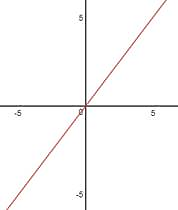Graph of f(x)=x2f(x)=x2
A function given by f(x)=x2f(x)=x2 is called the square function.
Y=x2y=x2 is a parabola and it’s also an even function, so it is symmetrical about the y-axis as shown in the figure: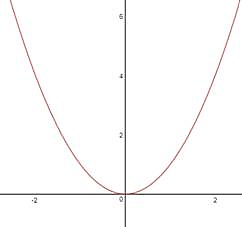Graph of f(x)=x3f(x)=x3

A function given by f(x)=x3f(x)=x3  is called the cube function. When a function is an odd function, its graph is symmetrical about the opposite quadrant or we can say that the graph is symmetrical about the origin as shown in the figure: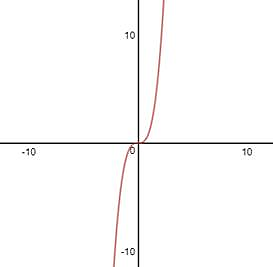On analyzing and extending the logic of the above three graphs, we can generalize the following:

Graph of f(x)=x2nf(x)=x2n; Where n is a natural number.

This will always be an even function.  Hence, the graph of f(x)=x2nf(x)=x2n will always be symmetrical about the y-axis.

The below figure shows graphs of the function for some values of n.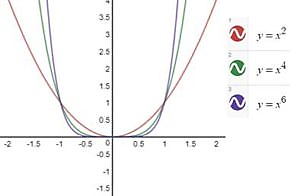Graph of f(x)=x2n–1f(x)=x2n–1; Where n is a natural number.

This will always be an odd function.  Hence, the graph of f(x)=x2n–1f(x)=x2n–1  will always be symmetrical about the origin or opposite quadrants.

The below figure shows graphs of the function for some values of n.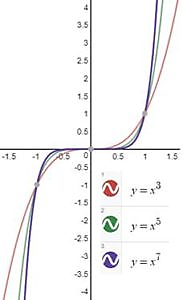Graphs of rational expressions

Rational functions are the functions obtained by dividing a polynomial by another polynomial, i.e. f(x)=P(x)Q(x)f(x)=P(x)Q(x)
In the CAT exam, we don’t get complicated rational expressions. Rather, we get simple rational expression functions, and their graphs are easy to plot as well.
Let us take some elementary rational functions and their corresponding graphs.
1. Graph of f(x)=1xf(x)=1x
This is also called the reciprocal function or a rectangular hyperbola. This is also an odd function, so its graph is symmetrical about the opposite quadrant.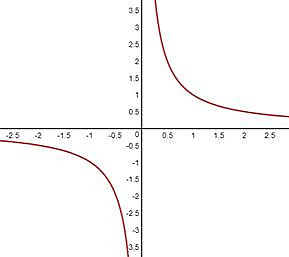1. Graph of f(x)=1x2f(x)=1×2
Since the power of x is even, clearly, this is an even function. So it must be symmetrical about the y-axis. Also, even negative values of the function f(x) will always be positive.  It is also important to mention here that the domain of the function will be all real values except 0.  that is, it cannot take the value 0.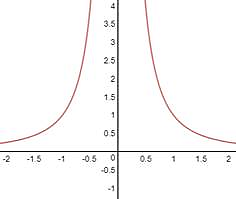Absolute value function (or modulus function):
• y=|x|={x;x≥0x;   x<0y=|x|={x;x≥0x;   x<0 .It is a numerical value of x
• It is symmetrical about y axis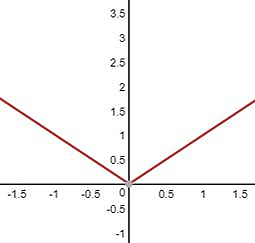Properties of modulus functions:
• |a|≤b ⇒–b ≤a≤ b;(a≥) |a|≤b⇒–b ≤a ≤b;(b≥0)
• |a|≥b⇒ a ≤–bora≥b;(b≥0) |a|≥ b ⇒ a≤–bora≥b;(b≥0)
• |x ± y|≥||x|–|y|||x ±y|≥||x|–|y||
Exponential function
The function f(x)=axf(x)=ax, where a≠0a≠0, and x is a real number, is called exponential function.
Depending upon the value of a, we can have all the increasing function or decreasing function.
Case I: If a >1
Say, f(x)=y=4xf(x)=y=4x increases with the increase in x.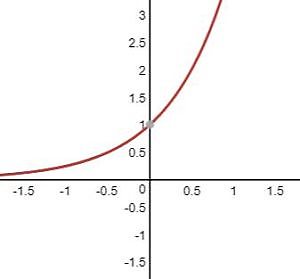Case II: If 0< a < 1
Say, f(x)=y = (12) xf(x)=y= (12)x  decreases with an increase in x.
In general, exponential function increases or decreases as (a>1) or (0<a<1) respectively.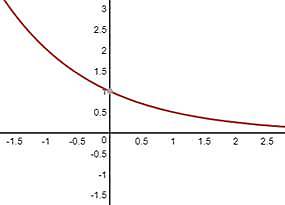Logarithmic function
This is an inverse of an exponential function. In other words, in the exponential function if we replace x with y and y with x, and rearrange the function taking x as the independent variable and as the dependent variable, then the new function will be a logarithmic function.
The logarithmic function is defined as f(x)=logax;(x,a>0)f(x)=logax;(x,a>0) and a≠0a≠0. The domain of logarithmic function is all positive real number.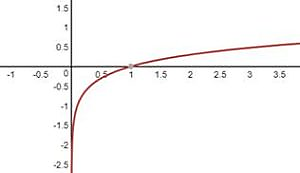These are the graphs of basic functions that frequently appeared in the CAT exam.

## CAT Quantitative Aptitude questions | CAT Algebra : Functions

Some of the important CAT questions on number system that appeared in the previous papers are: –

CAT 2020-

1. If f(5 + x) = f(5- x) for every real x, and f(x) = 0 has four distinct real roots, then the sum of these roots is

A) 10           B) 0 C) 40     D) 20

If f(5 + x) = f(5- x) for every real x, and f(x) = 0 has four distinct real roots, then the sum of these roots is

A) 10           B) 0 C) 40     D) 20

Given,

f(5-x) = f(5+x)

Put (5 +x) = k or -x = 5 –k

So f(5 +5 -k ) = f(k)

F(10-k) = f(k)

From this we can say if (k) = 0 then f(10-k) =0

Or if k is one root then 10-k will be other root and if x is 3rd root the 10-x will be fourth root thus sum of roots = k + 10-k + x+10-x = 20

2.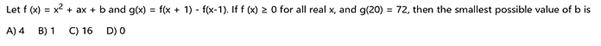Given, g(x) = f(x+1) – f(x-1)

g(x) = (x+1)^2 + a(x+1) +b – (x-1)^2 – a(x-1) – b

g(x) = 4x +2a

Also ,

g(20) = 72

4*20 + 2a = 72

Or a = -4

Now as f(x) >=0

It means (4ac-b^2)/4a≥0

(4b-a^2)/4≥0

Or (4b-16)/4≥0 ot b≥4 So least value of b =4

3. If f(x + y) = f (x) f (y) and f(5) = 4, then f (10) – f (-10) is equal to

A) 14.0625      B) 3 C) 0    D) 15.9375

We are told that f(x+y) = f(x) × f(y)

f(x) = f(x + 0) = f(x) × f(0)

f(x) = f(x) × f(0)

Assuming that f(x) is non-zero;

This implies: f(0) = 1

CAT 2019-

4. Consider a function f satisfying f (x + y) = f (x) f ( y) where x, y are positive integers, and f (1) = 2. If f (a +1) + f (a + 2) +…+ f (a + n) = 16(2n  −1) then a is equal to ________ .

Given, f(x+y) = f(x)*f(y), and f(1) =2

f(a+1) + f(a+2) + f(a+3) + …..+f(a+n) = 16 (2^n -1)————–1)

putting n =1

f(a+1) = 16

f(a)*f(1) = 16

or f(a) = 8 as f(1) =2

Putting n =2 in eq 1)

F(a+1) + f(a+2) =16*(2^2 -1)

16 + f(a)*f(2) = 16*3 = 48

8*(f2) = 48 -16 = 32

Or f(2) = 4

By putting n = 3 , in eq 1 we get,

f(a+1) + f(a+2) + f(a+3) = 16*(2^3 -1)

16 + 32 + f(a)*f(3) = 16*7 = 112

F(a)*f(3) = 112 – 16 – 32 = 64

8*f(3) = 64

F(3) = 8

As we know f(a) = 8 so a = 3

## How to deal with that topic preparation

Functions form an important part of Arithmetic which has a good contribution in the Quants section of CAT, XAT, SNAP, NMAT, and other various management entrance exams. To cope with the preparation of this topic is divided into three Level followings which will help the aspirants to score exceptionally well in the management entrance exams.

Level I:

In Phase I the candidate can start with basic concept understanding and should try solving the basic CAT questions from the MBAP CAT E Book (Concept Theory) study material and MBAP Live Lecture Recording (Basic) on basic concept. For this aspirants can follow Arun Sharma’s book and videos on Quantitative aptitude and after following the concept can solve the CAT questions s under Level of Difficulty 1 from the topic using MBAP CAT E Book (Practise Questions) and MBAP lecture Assignment

Level II:

In Phase II aspirants can start practicing CAT questions from Level of difficulty 2 questions from Arun Sharma book and videos from the given topic Function and also practice through the sectional test as time-based practice and analysis of the test is also necessary by this time using MBAP Topic wise Previous Year CAT Question

Level III:

In Phase III aspirants can practice these topics from previous year papers of prominent management entrance exams like CAT, XAT, SNAP, NMAT, and various other exams using MBAP Previous year CAT paper. Aspirants can also practice through a mock test and analyze it thoroughly. Also, in this phase aspirants should go through the concepts once again at the same time should try solving advanced problems from the Function from MBAP CAT Advance E books.Counselling Session
By IIM Mentor

#### Words From Our StudentsNihar Mehta
Calls from IIMs, SPJIMR & MDI
During my CAT preparation, I enrolled at MBA Pathshala for classroom coaching. Abhijit Sir & Haider Sir guided me through the process of planning & executing the strategies I had to use to succeed in the test. I received regular feedback on my progress during the GD and PI preparations after CAT. I received calls from IIMs, SPJIMR, and MDI. MBAP played a huge role in this, and I cannot thank them enough for their efforts.Ishan Rathod
Calls from IIM I, S, and all New And Baby IIM's.
MBAP is a great place to prepare for MBA-CET, CAT, and CMAT, and the faculty are unbeatable. Most importantly, they pay personal attention to each student. Most faculty members come from IIMs. One-on-one interaction helped me improve as the exams approached, as well as get calls from IIMs I, S, and all New and Baby IIMs. Interview sessions were very beneficial for me to convert IIM S.Sanskriti Reja
Calls from IIMs, XLRI, XIMB
I am glad I chose MBA Pathshala for my MBA coaching. The faculty here is excellent and very competent. If I had any questions or needed extra classes, they always gave me one. I got through my GD-PIs too after the exams with their help. Thanks to MBAP, I was able to pass CAT and other entrance exams. Among others, I received calls from XLRI, XIMB, and IIMs. A few of the colleges I've converted are KJ Somaiya, TAPMI, IIM Sambalpur, and IIM Sirmaur.Parth Kanakia
Converted SPJIMR
MBAP prepared me for GD, WAT, and PI. Abhijit Singh, Haider, and other MBAP faculty provided excellent training. Good knowledge was imparted from the very beginning. Regular mock interviews were conducted. As a former SPJIMR, I would recommend students to join MBAP.Ronit jaiswal
Calls from IIM-A/B/C & SNAP 98.12%ile
Coaching offers me personalized attention and a competitive environment. Because the preparation was so rigorous, I eventually began to perform at my best. The mock interviews/GD/WATs were conducted by anonymous panels, and it really boosted my confidence. Although I got a call from SCMHRD (SNAP-98.12), Abhijeet sir felt that I should try again and aim for IIM-A/B/C. It has been your pleasure to have been your mentor.Maitreya Khanapurkar
Calls From IIMs
You will get good support from the faculty until you get into a B-School. The shortcut techniques you will learn will be extremely useful. You will be able to resolve doubts at any time. If you plan on taking CAT CET or any other entrance exam, go for MBA Pathshala. With the help of MBAP tutors, I received a call from IMT Ghaziabad.
Yash Dandavate
GMAT Score - 660
GMAT prep with MBAP was the best I've ever experienced. With Rohan Kulkarni Sir's Verbal Guidance, I scored 85th percentile in my GMAT exam as I was very weak in English. I always found Haider Sir and Abhijeet to answer my questions and to develop strategies and shortcuts to tackle GMAT problems for me. MBAP is a great option for GMAT and CAT prep.

#### Free Material Area